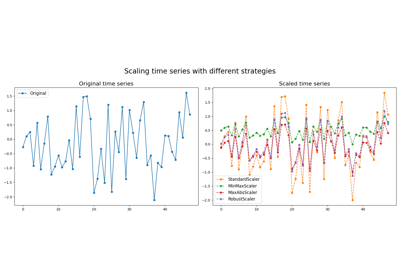# `pyts.preprocessing`.StandardScaler¶

class `pyts.preprocessing.``StandardScaler`(with_mean=True, with_std=True)[source]

Standardize time series by removing mean and scaling to unit variance.

Parameters: with_mean : bool (default = True) If True, center the data before scaling. with_std : bool (default = True) If True, scale the data to unit variance.

Examples

```>>> from pyts.preprocessing import StandardScaler
>>> X = [[0, 2, 0, 4, 4, 6, 4, 4],
...      [1, 0, 3, 2, 2, 2, 0, 2]]
>>> scaler = StandardScaler()
>>> scaler.transform(X)
array([[-1.5, -0.5, -1.5,  0.5,  0.5,  1.5,  0.5,  0.5],
[-0.5, -1.5,  1.5,  0.5,  0.5,  0.5, -1.5,  0.5]])
```

Methods

 `__init__`([with_mean, with_std]) Initialize self. `fit`([X, y]) Pass. `fit_transform`(X[, y]) Fit to data, then transform it. `get_params`([deep]) Get parameters for this estimator. `set_params`(**params) Set the parameters of this estimator. `transform`(X) Perform standardization by centering and scaling.
`__init__`(with_mean=True, with_std=True)[source]

Initialize self. See help(type(self)) for accurate signature.

`fit`(X=None, y=None)[source]

Pass.

Parameters: X Ignored y Ignored self : object
`fit_transform`(X, y=None, **fit_params)

Fit to data, then transform it.

Fits transformer to X and y with optional parameters fit_params and returns a transformed version of X.

Parameters: X : array-like, shape = (n_samples, n_timestamps) Univariate time series. y : None or array-like, shape = (n_samples,) (default = None) Target values (None for unsupervised transformations). **fit_params : dict Additional fit parameters. X_new : array Transformed array.
`get_params`(deep=True)

Get parameters for this estimator.

Parameters: deep : bool, default=True If True, will return the parameters for this estimator and contained subobjects that are estimators. params : dict Parameter names mapped to their values.
`set_params`(**params)

Set the parameters of this estimator.

The method works on simple estimators as well as on nested objects (such as `Pipeline`). The latter have parameters of the form `<component>__<parameter>` so that it’s possible to update each component of a nested object.

Parameters: **params : dict Estimator parameters. self : estimator instance Estimator instance.
`transform`(X)[source]

Perform standardization by centering and scaling.

Parameters: X : array-like, shape = (n_samples, n_timestamps) Data to scale. X_new : array-like, shape = (n_samples, n_timestamps) Scaled data.

## Examples using `pyts.preprocessing.StandardScaler`¶Scalers

Scalers